## 20110617

### Sketchbook: Japantown Waifer X110530-1230852
http://www.flickr.com/photos/waiferx/5779563951/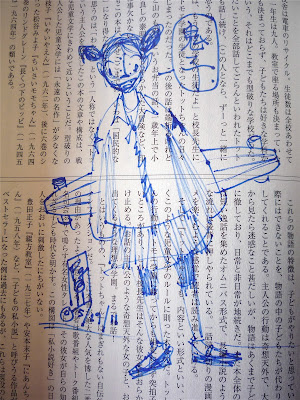110530-1230853
http://www.flickr.com/photos/waiferx/5779564129/

Waifer X Industries, Inc. cartoon character Waifer X, from the 1997-2002 webcomic "Did you know? by NK Particle" (cf. http://waiferx.com/Cartoons/archivewindow.html). Ink on found Japantown newsletter, San Francisco, CA, (c) 2011 Patrick M. Len.

## 20110616

### Kudos: fantastic semester"Fantastic semester..." by Jennifer R.
Astronomy 210
May 2011
Cuesta College, San Luis Obispo, CA

## 20110615

### Student scribble: "P-dog"110131-Pdog
http://www.flickr.com/photos/waiferx/5762866856/

Doodle by E. F. Ontiveros of Cuesta College Physical Sciences Division instructor Dr. Patrick M. Len. Astronomy 210 lecture, Cuesta College, San Luis Obispo, CA.

## 20110614

### Kudos: rave review110516-card2
http://www.flickr.com/photos/waiferx/5762335781/110516-card1a
http://www.flickr.com/photos/waiferx/5762871858/

"Rave Review," Physics 205B-30882 students, Cuesta College, San Luis Obispo, CA. Thank-you card. Like a grade-school valentine, ran by and tossed on my desk, hoping nobody saw it just happen.

## 20110613

### Astronomy final exam question: Drake equation as "sound science?"

Astronomy 210 Final Exam, Spring Semester 2011
Cuesta College, San Luis Obispo, CA

H*bent...: Do you think that the Drake [e]quation is just a bunch of wishful thinking?
Keith P: No, the equation is sound science...
Discuss whether or not if this answer is correct, and how you know this. Explain using the properties of the Drake equation, and the factors used in the Drake equation.

• p = 20/20:
Correct. Understands what the Drake equation calculates, and the general trend of known, general quantities to less known, specific quantities that are plugged into it. Then makes an argument as to whether this is scientific (e.g., as a methodical process, even though it is incomplete) or non-scientific (e.g., based on speculation of untestable, nonfalsifiable concepts).
• r = 16/20:
Nearly correct (explanation weak, unclear or only nearly complete); includes extraneous/tangential information; or has minor errors.
• t = 12/20:
Contains right ideas, but discussion is unclear/incomplete or contains major errors. As (p), but does not discuss criteria for deciding solid science versus wishful thinking, or does not adequately discuss the Drake equation.
• v = 8/20:
Limited relevant discussion of supporting evidence of at least some merit, but in an inconsistent or unclear manner.
• x = 4/20:
Implementation/application of ideas, but credit given for effort rather than merit.
• y = 2/20:
Irrelevant discussion/effectively blank.
• z = 0/20:
Blank.
Section 30674
Exam code: finaln4St
p: 17 students
r: 0 students
t: 15 students
v: 4 students
x: 0 students
y: 0 students
z: 0 students

Section 30676
Exam code: finalS45s
p: 18 students
r: 0 students
t: 8 students
v: 11 students
x: 4 students
y: 2 students
z: 0 students

A sample "p" response (from student 0920):Another sample "p" response (from student 9001):A sample "v" response (from student 5259):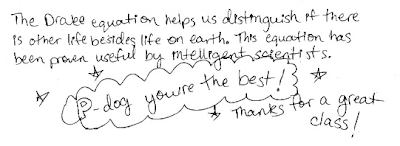A sample "x" response (from student 2221), who at least recalls that the Drake equation has "SWAG" (scientific wild-a\$\$ guesses) in its component factors:A sample "y" response (from student 1992):## 20110612

### Astronomy final exam question: Earth avoiding Venus' runaway greenhouse?

Astronomy 210 Final Exam, spring semester 2011
Cuesta College, San Luis Obispo, CA

Samantha: How did Earth avoid the runaway greenhouse effect that made Venus so hot?
Paul: The reason that Earth managed to avoid a catastrophic runaway greenhouse effect was its level of geologic activity... Venus, on the other hand, is a geologically inactive planet, with no way of recycling [carbon dioxide].
Discuss whether or not if this answer is correct, and how you know this. Explain using the properties of greenhouse gases and geological activity.

• p = 20/20:
Correct. Understands how oceans absorb carbon dioxide (which eventually gets subducted into the mantle), and specifically discusses how Earth avoided runaway greenhouse effect by being farther away from the sun than Venus.
• r = 16/20:
Nearly correct (explanation weak, unclear or only nearly complete); includes extraneous/tangential information; or has minor errors. As (p), but does not discuss how location was key for Earth to avoid runaway greenhouse effect as on Venus.
• t = 12/20:
Contains right ideas, but discussion is unclear/incomplete or contains major errors. At least recognizes factors that contribute to and/or control greenhouse effect.
• v = 8/20:
Limited relevant discussion of supporting evidence of at least some merit, but in an inconsistent or unclear manner. Discusses factors that do not contribute to and/or control greenhouse effect.
• x = 4/20:
Implementation/application of ideas, but credit given for effort rather than merit.
• y = 2/20:
Irrelevant discussion/effectively blank.
• z = 0/20:
Blank.
Section 30674
Exam code: finaln4St
p: 12 students
r: 7 students
t: 8 students
v: 2 students
x: 0 students
y: 0 students
z: 1 student

Section 30676
Exam code: finalS45s
p: 10 students
r: 12 students
t: 8 students
v: 12 students
x: 0 students
y: 0 students
z: 1 student

A sample "p" response (from student 1440):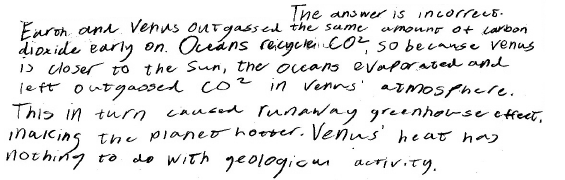A sample "r" response (from student 0701):## 20110611

### Astronomy final exam question: finding age of a star cluster from its colors

Astronomy 210 Final Exam, spring semester 2011
Cuesta College, San Luis Obispo, CA

An astronomy question on an online discussion board[*] was asked and answered:
Sng: What is the easiest way to determine the age of a star cluster?
qcp: If a star cluster has a lot of white/blue stars, it's a young cluster. If it contains fewer white stars, but more yellow stars, it's older.
Discuss whether or not if this answer is correct, and how you know this. Explain using the properties of mass and stellar lifetimes, evolution of stars, and star cluster ages.

• p:
Correct. Understands that massive stars evolve faster than medium-mass stars, such that massive stars reach the upper left of the main sequence first, (becoming "blue/white stars"), spend the least amount of time there, and leave before medium-mass stars become main-sequence ("yellow") stars would in an older star cluster. May instead interpret statement as incorrect, if "white stars" is taken to mean white dwarfs, which would be in older star clusters than those with medium-mass main sequence stars.
• r:
Nearly correct (explanation weak, unclear or only nearly complete); includes extraneous/tangential information; or has minor errors. May instead discuss how the metallicity can be used to find the age of a star cluster (as being "easier" than comparing colors of (main) sequence stars).
• t:
Contains right ideas, but discussion is unclear/incomplete or contains major errors. At least understands how massive and low-mass stars evolve at different rates to get to (and leave) the main sequence.
• v:
Limited relevant discussion of supporting evidence of at least some merit, but in an inconsistent or unclear manner.
• x:
Implementation/application of ideas, but credit given for effort rather than merit.
• y:
Irrelevant discussion/effectively blank.
• z:
Blank.
Section 30676
Exam code: finalS45s
p: 8 students
r: 4 students
t: 15 students
v: 14 students
x: 1 student
y: 0 students
z: 1 student

A sample "p" response (from student 8487):Another sample "p" response (from student 0701), appealing to the "House Party" model of star cluster age and stellar evolution: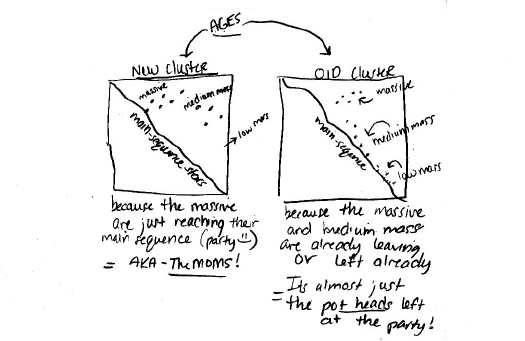A sample "p" response (from student 9936), interpreting "white stars" as white dwarfs instead of white-hot massive main sequence stars: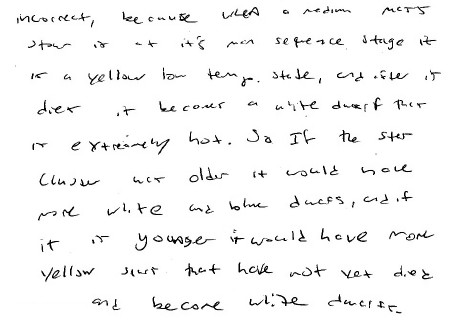## 20110610

### Astronomy final exam question: main-sequence star colors vs. sizes

Astronomy 210 Final Exam, Spring Semester 2011
Cuesta College, San Luis Obispo, CA

Jayen: While looking at the H-R diagram I noticed the [bluer] stars were bigger, and the [redder] stars were smaller. Is this always the case [for] stars in the main sequence?
lithiumdeuteride: Not necessarily...
Discuss whether or not if this answer is correct, and how you know this. Explain using Wien's law, the Stefan-Boltzmann law and/or an H-R diagram.

• p = 20/20:
Correct. Wien's law: blue stars are hotter, and are located on the left side of an H-R diagram, while red stars are cooler, and are located on the right side. Interprets H-R diagram to show that for main sequence stars, blue stars are larger than red stars.
• r = 16/20:
Nearly correct (explanation weak, unclear or only nearly complete); includes extraneous/tangential information; or has minor errors.
• t = 12/20:
Contains right ideas, but discussion is unclear/incomplete or contains major errors. Applies Wien's law, but does not limit scope of discussion to main sequence stars.
• v = 8/20:
Limited relevant discussion of supporting evidence of at least some merit, but in an inconsistent or unclear manner. At least attempts to use Wien's law, H-R diagram and/or the Stefan-Boltzman law.
• x = 4/20:
Implementation/application of ideas, but credit given for effort rather than merit. Discussion not based on Wien's law, H-R diagram and/or the Stefan-Boltzman law.
• y = 2/20:
Irrelevant discussion/effectively blank.
• z = 0/20:
Blank.
Section 30674
Exam code: finaln4St
p: 12 students
r: 7 students
t: 8 students
v: 4 students
x: 4 students
y: 1 student
z: 0 students

A sample "p" response (from student 7924):## 20110609

### Physics final exam problem: Acerinox Cs-137 contamination

Physics 205B Final Exam, spring semester 2011
Cuesta College, San Luis Obispo, CA

Cf. Giambattista/Richardson/Richardson, Physics, 2/e, Problem 29.38

In May 1998, the Acerinox steel factory[*] in Spain accidentally recycled material containing radioactive (137,55)Cs, such that 1 gram of contaminated ashes[**] were found to have as high as 1,420 decays per second. Suppose that in May 2011, this same 1 gram sample of ashes now has 1,050 decays per second. What is the half-life of ￼cesium-137, assuming that it is the only source of radiation in this sample? Show your work and explain your reasoning.

[**] www10.antenna.nl/wise/495/4895.html.

• p:
Correct. Sets up exponential or half-life exponent decay formula to solve for half-life T1/2, given R0 and R, and t = 13 years.
• r:
Nearly correct, but includes minor math errors.
• t:
Nearly correct, but approach has conceptual errors, and/or major/compounded math errors.
• v:
Implementation of right ideas, but in an inconsistent, incomplete, or unorganized manner.
• x:
Implementation of ideas, but credit given for effort rather than merit.
• y:
Irrelevant discussion/effectively blank.
• z:
Blank.

Section 30882
Exam code: finalv0L7
p: 3 students
r: 3 students
t: 0 students
v: 1 student
x: 0 students
y: 1 student
z: 0 students

A sample "p" response (from student 2180), using number of radioactive nuclei (N) instead of activity (R), but still essentially correct:## 20110608

### Physics final exam problem: voltmeter reading

Physics 205B Final Exam, Spring Semester 2011
Cuesta College, San Luis Obispo, CA

Cf. Giambattista/Richardson/Richardson, Physics, 2/e, Problem 18.65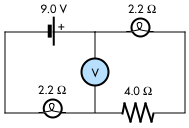[20 points.] An ideal 9.0 V emf battery is connected to an ideal voltmeter, a 4.0 Ω resistor and two 2.2 Ω light bulbs, as shown at right. What is the reading of the voltmeter? Show your work and explain your reasoning.

• p = 20/20:
Correct. Finds equivalent resistance of series circuit to find current, then applies some part of Kirchhoff's voltage rule to determine difference in voltage, either using the left part of the circuit (delta(V) = |-(2.2 ohms)*I + (9.0 V)|), or the right part (delta(V) = |–I*(2.2 ohms + 4.0 ohms)|).
• r = 16/20:
Nearly correct, but includes minor math errors.
• t = 12/20:
Nearly correct, but approach has conceptual errors, and/or major/compounded math errors.
• v = 8/20:
Implementation of right ideas, but in an inconsistent, incomplete, or unorganized manner. Problematic application of equivalent resistance rules and Kirchhoff's rules.
• x = 4/20:
Implementation of ideas, but credit given for effort rather than merit.
• y = 2/20:
Irrelevant discussion/effectively blank.
• z = 0/20:
Blank.

Section 30882
Exam code: finalv0L7
p: 1 student
r: 2 students
t: 3 students
v: 1 student
x: 0 students
y: 1 student
z: 0 students

A sample "p" response (from student 7503):## 20110607

### Physics final exam problem: two lens system

Physics 205B Final Exam, Spring Semester 2011
Cuesta College, San Luis Obispo, CA

Cf. Giambattista/Richardson/Richardson, Physics, 2/e, Problem 24.5

[20 points.] An upright object is located 14.0 cm in front of a diverging lens with focal length –10.0 cm. Behind the diverging lens is a converging lens of focal length +16.0 cm. (Drawing below is not to scale.) Find the minimum separation distance between the diverging lens and converging lens such that the final image will be inverted, with respect to the original object. Show your work and explain your reasoning.• p = 20/20:
Correct. Determines that the first diverging lens will produce an upright (virtual) image located 5.8 cm in front of it. For the second converging lens to produce an inverted (real) image, its object must be located at least 16.0 in front of it. Since the image from the first lens (5.8 cm in front of it) will be the object for the second lens, the second lens must be placed at a minimum distance of 10.2 cm behind the first lens in order for the second lens to produce a final inverted (real) image.
• r = 16/20:
Nearly correct, but includes minor math errors. May have not sufficiently explained the conditions for the second lens to create an inverted (real) image in terms of its object and focal length positions.
• t = 12/20:
Nearly correct, but approach has conceptual errors, and/or major/compounded math errors. At least determined the location of the image produced by the first lens, and some attempt at constraining the location of the second lens.
• v = 8/20:
Implementation of right ideas, but in an inconsistent, incomplete, or unorganized manner.
• x = 4/20:
Implementation of ideas, but credit given for effort rather than merit.
• y = 2/20:
Irrelevant discussion/effectively blank.
• z = 0/20:
Blank.

Section 30882
Exam code: finalv0L7
p: 2 students
r: 1 student
t: 2 students
v: 2 students
x: 0 students
y: 1 student
z: 0 students

A sample "p" response (from student 2414):## 20110606

### Physics final exam question: slide-wire generator

Physics 205B Final Exam, spring semester 2011
Cuesta College, San Luis Obispo, CA

Cf. Giambattista/Richardson/Richardson, Physics, 2/e, Problem 20.5A conducting rod in a uniform external magnetic field slides on top of metal rails, which are connected to a light bulb to form a complete circuit. Determine the direction of the current (clockwise or counterclockwise) through the circuit if the rod is moved to the right at constant speed. Explain your reasoning using the properties of circuits, right-hand rules, Faraday's law and/or Lenz's law.

• p:
Correct. Applies RHR2 to determine direction of force on positive charges in moving rod, which results in a counterclockwise current in the loop. Or uses Faraday's law and Lenz's law to determine that the increasing flux through the loop into the page must be counteracted, and uses RHR3 to determine the direction (counterclockwise) of the induced current in the loop.
• r:
As (p), but argument indirectly, weakly, or only by definition supports the statement to be proven, or has minor inconsistencies or loopholes. May have direction/sign wrong in RHR2/flux/F's law/L's law/RHR3 process.
• t:
Nearly correct, but argument has conceptual errors, or is incomplete. Few missing pieces, but still demonstrates RHR2/flux/F's law/L's law/RHR3 process.
• v:
Limited relevant discussion of supporting evidence of at least some merit, but in an inconsistent or unclear manner.
• x:
Implementation/application of ideas, but credit given for effort rather than merit.
• y:
Irrelevant discussion/effectively blank.
• z:
Blank.

Section 30882
Exam code: finalv0L7
p: 5 students
r: 0 students
t: 1 student
v: 1 student
x: 0 students
y: 1 student
z: 0 students

A sample "p" response (from student 9904):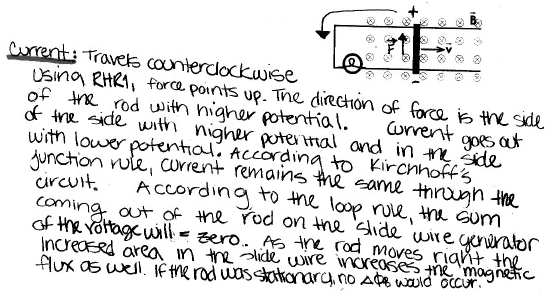## 20110605

### Physics final exam question: electric field of two charges

Physics 205B Final Exam, Spring Semester 2011
Cuesta College, San Luis Obispo, CA

Cf. Giambattista/Richardson/Richardson, Physics, 2/e, Problem 16.32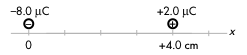[10 points.] Two point charges are fixed at the locations shown at right. Determine the direction of the total electric field at the location x = +3.0 cm. Explain your answer using the properties of charges, electric fields, and superposition.

• p = 10/10:
Correct. At x = +3.0 cm, electric field of -8.0 µC charge points to the left, while electric field of 2.0 µC charge also points to the left, such that the total electric field at that location points to the left.
• r = 8/10:
As (p), but argument indirectly, weakly, or only by definition supports the statement to be proven, or has minor inconsistencies or loopholes. Chooses stronger electric field contribution to determine direction of total electric field.
• t = 6/10:
Nearly correct, but argument has conceptual errors, or is incomplete. Calculates magnitudes of each electric field contribution at x = +3.0 cm, but garbles directions and/or superposition.
• v = 4/10:
Limited relevant discussion of supporting evidence of at least some merit, but in an inconsistent or unclear manner.
• x = 2/10:
Implementation/application of ideas, but credit given for effort rather than merit.
• y = 1/10:
Irrelevant discussion/effectively blank.
• z = 0/10:
Blank.

Section 30882
Exam code: finalv0L7
p: 3 students
r: 2 students
t: 3 students
v: 0 students
x: 0 students
y: 0 students
z: 0 students

A sample "p" response (from student 3773):## 20110604

### Physics quiz question: relativistic electrons

Physics 205B Quiz 7, Spring Semester 2011
Cuesta College, San Luis Obispo, CA

Cf. Giambattista/Richardson/Richardson, Physics, 2/e, Problem 26.45

If electrons had a Lorentz factor γ = 2.00, how fast would they be traveling?
(A) 0.414c.
(B) 0.500c.
(C) 0.866c.
(D) 1.41c.

Inverting the Lorentz factor equation γ = 1/sqrt(1 - (v/c)^2)) to solve for v results in:

v = c*sqrt(1 - 1/(γ^2)),

such that with γ = 2.00, v = 0.886c. Response (A) is 1 - c*sqrt(2*(γ - 1)); response (B) is c*sqrt(1/(γ^2)); response (D) is c*sqrt(2*(γ - 1)), which results in an implausible result.

Section 30882
Exam code: quiz07t0LO
(A) : 0 students
(B) : 1 student
(C) : 5 students
(D) : 2 students

"Success level": 75%
Discrimination index (Aubrecht & Aubrecht, 1983): -0.50

Compare to an older version of this question from Fall 2010.

## 20110602

### Physics quiz question: radioactive decay age

Physics 205B Quiz 7, Spring Semester 2011
Cuesta College, San Luis Obispo, CA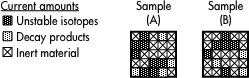Consider two samples with differing amounts of radioactive elements, embedded gaseous daughter elements, and inert material (which is not involved in the decay process). Which sample is older, as determined by radioactive dating?
(A) Sample A.
(B) Sample B.
(C) (There is a tie.)
(D) (Cannot be determined, as they have different amounts of inert material.)

The solidification age of a sample (how long ago has it been since it was last molten) is determined by the ratio of decay products to its unstable isotopes. A larger ratio of decay products to unstable isotopes corresponds to a sample with a very old solidification age, but since both samples have the same ratio of decay products to unstable isotopes, they must have the same solidification age.

Section 30882
Exam code: quiz07t0LO
(A) : 4 students
(B) : 1 student
(C) : 3 students
(D) : 0 students

"Success level": 38%
Discrimination index (Aubrecht & Aubrecht, 1983): 0

Related post:
• Astronomy quiz question: radioactive decay age.
• ## 20110601

### Physics quiz archive: radioactive decay, special relativity

Physics 205B Quiz 7, Spring Semester 2011
Cuesta College, San Luis Obispo, CA

Section 30882, version 1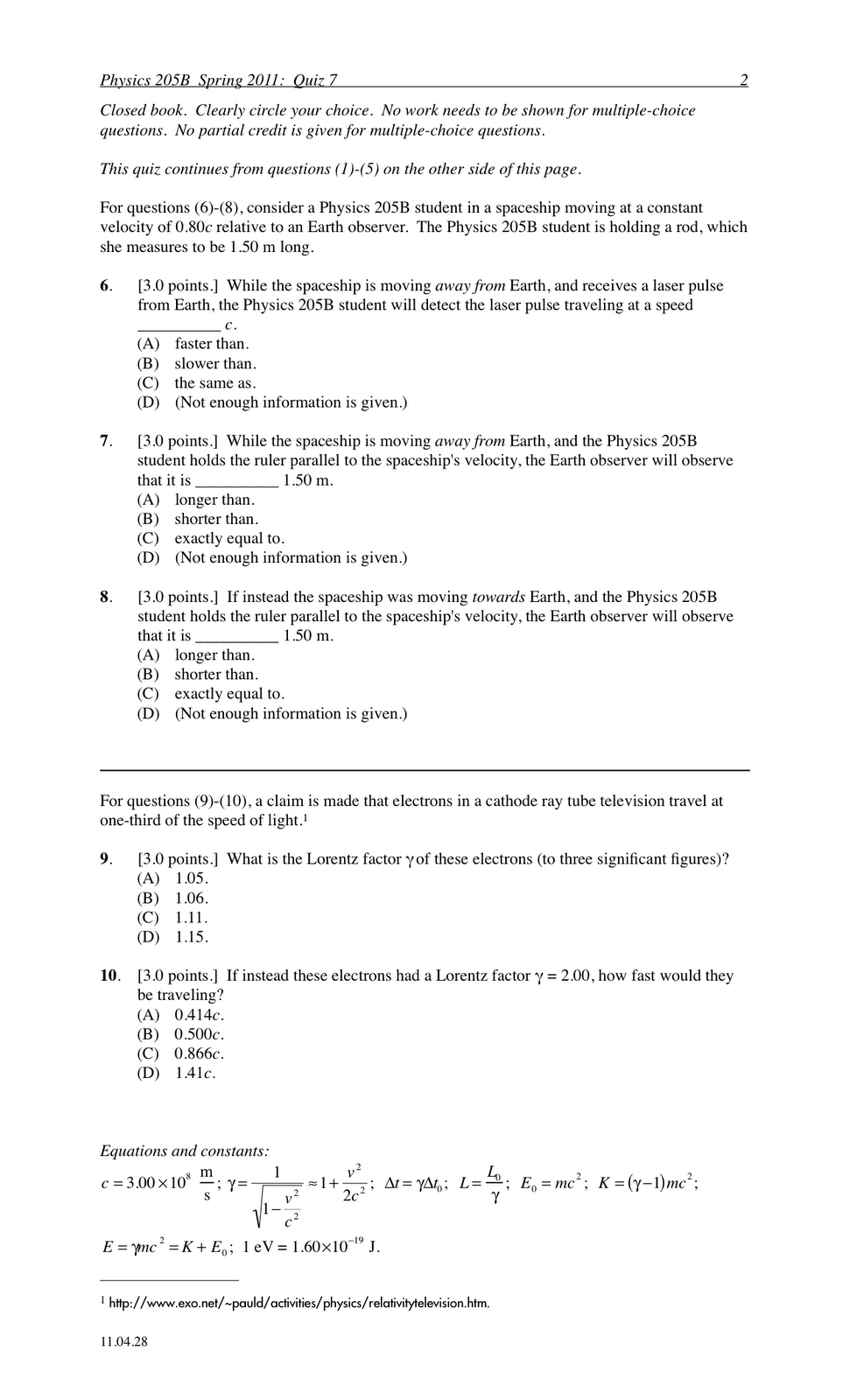Section 30882 results
Exam code: quiz07t0LO
``` 0- 6 :
7-12 :
13-18 : **  [low = 18]
19-24 : *****  [mean = 22.5 +/- 3.6]
25-30 : **  [high = 27]```